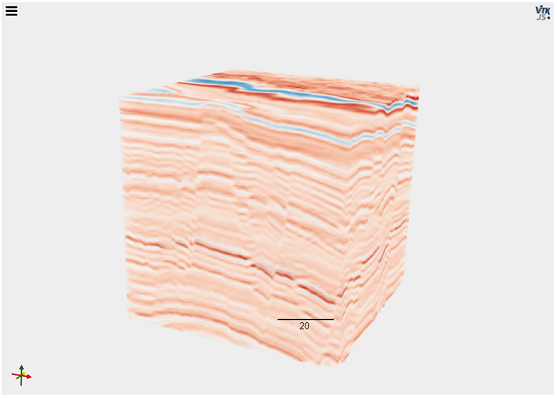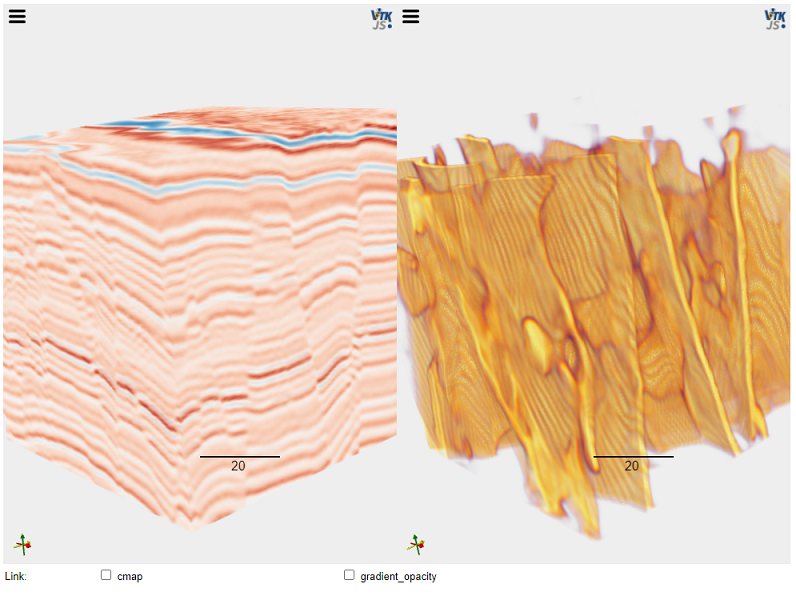# Cegal Prizm Python Tool Pro - Fault prediction#

## Enabling data science against Petrel#

In this Notebook, we show how you can use Python Tool Pro to apply complex machine learning algorithms on your Petrel data. In this case, we will use a neural network to predict a fault model on a seismic cube.

### Python libraries#

For this Notebook to work additional Python libraries are needed. The next code cell only needs to be executed if libraries are not installed in your Python environment.

[ ]:

# uncomment the lines of the Python libraries you need to install
# !pip install tensorflow
# !pip install matplotlib
# !pip install itkwidgets
# !pip install ipywidgets

:

from cegalprizm.pythontool import PetrelConnection
import matplotlib.pyplot as plt
import numpy as np
import tensorflow as tf
import matplotlib
import numpy as np
from IPython.display import Markdown, Image
import itkwidgets
import ipywidgets as widgets
from itkwidgets.widget_viewer import Viewer
import math


## Connect to Petrel#

The first step is to establish a connection to Petrel. We will create a Python variable called ‘ptp’ which we will use for accessing the Petrel data.

:

ptp = PetrelConnection()

print(f'Currently open Petrel project is {ptp.get_current_project_name()}')

Currently open Petrel project is PTPDemo_2021.pet


## Select and visualize your input data#

:

w_input = widgets.Dropdown(
options=ptp.seismic_cubes,
#value=input_options,
description='Select input:',
disabled=False,layout={'width':'max-content'},
)
display(w_input)

:

cube=ptp.seismic_cubes[w_input.label]
cube

:

Seismic(petrel_name="OriginalSeismicInput")


To visualize our seismic data input, we are using the itkwidgets library. We will create a chunk of the seismic of dimensions 128x128x128. This size is used because the neural network algorithm in this specific case requires the input data to to have these dimensions. For visualization or other calculations, different size chunks can be created using chunk().

:

cube.retrieve_history()
cube.path

:

'Input/Seismic/Faultprediction/OriginalSeismicInput'

:

span = 127
x = int((cube.extent.i - 128)/2)
y = int((cube.extent.j - 128)/2)
z = int((cube.extent.k - 128)/2)
arr  = cube.chunk((x,x+span),(y,y+span),(z,z+span)).as_array()

itkwidgets.view(image=(np.rot90(arr, 1, (0,2))-arr.mean())/arr.std(),shadow=False, gradient_opacity=0.0, ui_collapsed=True, cmap=itkwidgets.cm.BuRd, opacity_gaussians= [[{'position': 0.5, 'height': 1, 'width': 0.5, 'xBias': 0.0, 'yBias': 2.0}]])


The image below represents the output of the previous cell, a visualization of the seismic cube using the itkwidgets library:## Applying a pre-trained neural network algorithm#

We can now apply a pre-trained neural network for fault prediction on our chunk of the seismic data input and are then display the resulting fault model next to our seismic data. The pretrained model used is called ‘fseg-70.hdf5’.

:

loaded_model = load_model("fseg-70.hdf5", custom_objects={'cross_entropy_balanced': tf.keras.losses.BinaryCrossentropy()})

def fault_attribute_calculator(arr):
arr = np.rot90(arr, 1, (0,2))
arr = (arr - arr.mean())/arr.std()
n1, n2, n3 = 128, 128, 128
gx = np.reshape(arr,(1,n1,n2,n3,1))
Y = Y.reshape((n1,n2,n3))
return np.rot90(Y, -1, (0,2))

faults = fault_attribute_calculator(arr)

1/1 [==============================] - 2s 2s/step

:

def fault_compare(image1, image2,link_cmap=False, link_gradient_opacity=False,**viewer_kwargs):
"""Compare two images by visualizing them side by side.
Visualization traits, e.g. the view mode, camera, etc., are linked
between the viewers. Optional trait linking can be enabled in widget's
user interface.
"""

viewer1 = Viewer(image=image1, shadow=False, gradient_opacity=0.0, ui_collapsed=True, cmap=itkwidgets.cm.BuRd, opacity_gaussians= [[{'position': 0.5, 'height': 1, 'width': 0.33, 'xBias': 0.0, 'yBias': 2.0}]])
# Collapse the second viewer's user interface by default.
if 'ui_collapsed' not in viewer_kwargs:
viewer_kwargs['ui_collapsed'] = True
cmap=itkwidgets.cm.inferno,
opacity_gaussians= [[{'position': 0.5, 'height': 1, 'width': 0.5, 'xBias': 1, 'yBias': 1.75}]])

def __init__(self, enable, name):
self.name = name
if enable:

def __call__(self, change):
if change.new:
else:

left_sidebar=viewer1,
center=None,
right_sidebar=viewer2,
pane_heights=[1, 6, 1])
return widget

fault_compare(np.rot90(arr, 1, (0,2)), np.rot90(faults, 1, (0,2)))


We can now apply the neural network to the seismic cube chunk we got before. The image below represents the output of the previous cell, showing the seismic cube on the left and the interpreted faults on the right:### Information about the neural network algorithm#

This machine learning algorithm is published and GitHub and can be found using following link #### xinwucwp/faultSeg